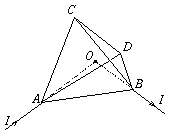Középiskolai Matematikai és Fizikai Lapok
Informatika rovattal
 Már regisztráltál? Új vendég vagy?# Exercises and problems in Physics December 2000

## New experimental problem:

M. 220. Prepare an air vane supported in good bearings using paper cones or table tennis balls cut in two. Measure how the number of revolutions per minute of the vane depends on the speed of the air current (wind). Can we deduce the coefficient of air resistance for the concave and the convex side using the measurement data?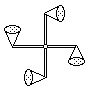## New problems:

P. 3384. Water is poured into a quadrangular vessel. What is the height of the vessel if the force exerted onto each side is greater than the weight of the water?

P. 3385. An aluminium cube with 2 dm sides and 2700 kg/m3 density is fastened to one end of a 3 m long chain lying on a table, each metre of which has a mass of 1 kg. The cube is lifted at a uniform speed of 0.5 m/s. What is the change of the potential energy after a) 5 seconds, b) 10 seconds of lifting?

P. 3386. A 3 litre container with insulated walls is divided into two equal parts by a rigid, heat conducting wall. There is oxygen with a pressure of 105 Pa and a temperature of 300 K in both parts: diatomic (O2) on the left side and triatomic (O3), i.e. ozone on the right. After some time, ozone passes into diatomic oxygen in such a way that two ozone molecules transform into three O2 molecules: 2O33O2. a) What is the number of oxygen molecules in each part? b) What are the pressure and temperature in each part when thermal equilibrium is reached if 2.4.10-19 J of energy are released during the transformation of each ozone molecule? (Consider the gases O3 and O2 as ideal gases of respectively 6 and 5 degrees of freedom.)

P. 3387. Why do children usually sweat less than adults when performing sports?

P. 3388. A body of mass m is slid upwards on a lath of inclination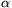in a uniform motion. Determine the magnitude and the direction of the minimum force required for the sliding. The coefficient of friction is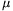.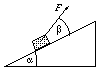P. 3389. Two bodies of respective masses m and M are joined to each other by a weightless thread flung over a pulley of negligible mass. The two bodies are held in the position shown in the figure and released at a given moment. Mass M is much (e.g. a thousand times) greater than mass m. Friction between the body of mass m and the table is negligible. Is the body of mass m separated from the table right after the two bodies are released?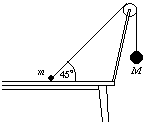P. 3390. What should the capacitance C in the circuit shown in the figure be so that the energy accumulating in it is maximum? What is this maximum amount of energy?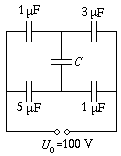P. 3391. An electron beam reaches the d=4 cm wide homogeneous magnetic field of induction B=0.02 T shown in the figure at an angle of incidence=30o, at right angles to vector B. a) Slow or fast particles can get through the magnetic field? b) At what pd. should the elctrons be accelerated so that they are reflected'' from the magnetic field?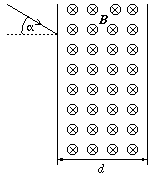P. 3392. In the series RLC circuit shown in the figure, the bulb shines. When the coil is short-circuited the light emission of the bulb does not change. How does it change when the capacitor is short-circuited?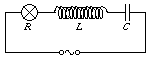P. 3393. A regular tetrahedron is made of a homogeneous resistance wire of uniform cross-section. Current I is conducted to vertex A through a long, straight piece of wire directed toward centre O of the tetrahedron, and it is conducted away from vertex B in the same fashion. What are the magnitude and the direction of the magnetic induction vector at the centre of the tetrahedron?